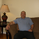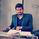1679 뷰
This script utilizes this concept. Instead of weighting by volume , it weights by amount of price action on every close price of the rolling window. I assume it can be used as an additional reference point for price mode and price antimode.

it is directly connected with Market (not volume ) profile, or TPO charts.

The algorithm:
1) takes a rolling window of, for example, 50 data points of close prices:
2) for each of this closing prices, the algorithm will check how many bars touched this close price.
3) then: sum of datapoints * weights/sum of weights

Since the logic is implemented in pretty non-efficient way, the script sometimes can take time to make calculations. Moreover, it calculates the centroid taking into account only close prices, not every tick. of a given rolling window That's why it's still experimental.
오픈 소스 스크립트

진정한 TradingView의 정신에 따라, 이 스크립트의 작성자는 스크립트를 오픈소스로 게시했기에 거래자들이 이해하고 확인할 수 있습니다. 작가님께 건배! 스크립트를 무료로 사용할 수 있지만, 게시물에서 이 코드를 재사용하는 것은 하우스룰을 따릅니다. 당신은 스크립트를 차트에 사용하기 위해 그것을 즐겨찾기 할 수 있습니다.

차트에 이 스크립트를 사용하시겠습니까?

## 코멘트I am assuming that in the pine_centroid function, x and y will be the close and the time?
Thanks.
응답syracusepro
@syracusepro, yes, take a look at the line 32 where the function is finally called. The third argument is "src", and the fourth is "length" .
응답gorx1
@gorx1, very interesting concept. can we modify this by having low and high, 2 centroid simultaneously instead of close. if yes, please share the script.
that will be highly appreciable.
응답syracusepro
@syracusepro, Ok, imagine you chose 100 close prices in script's settings. Now there is a function, I called it "density", on line 11. Now this function will take each of these 100 close prices and check how many bars touched each of these close prices within the rolling window. Hope it does make sense, if not, imma draw a picture n drop it here.
응답